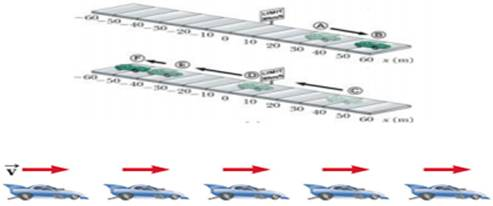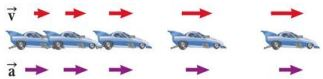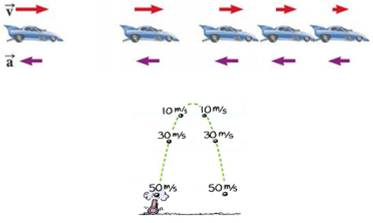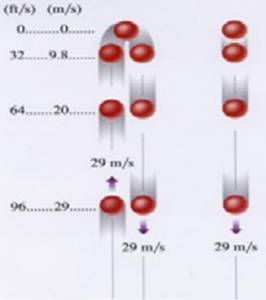# Motion class 9 Notes Science## myCBSEguide App

CBSE, NCERT, JEE Main, NEET-UG, NDA, Exam Papers, Question Bank, NCERT Solutions, Exemplars, Revision Notes, Free Videos, MCQ Tests & more.

CBSE class 9 Science Chapter 8 Motion notes in PDF are available for free download in myCBSEguide mobile app. The best app for CBSE students now provides Motion class 9 Notes latest chapter wise notes for quick preparation of CBSE exams and school based annual examinations. Class 9 Science notes on Chapter 8 Motion are also available for download in CBSE Guide website.

## 9 Science notes Chapter 8 Motion

Download CBSE class 9th revision notes for Chapter 8 Motion in PDF format for free. Download revision notes for Motion class 9 Notes and score high in exams. These are the Motion class 9 Notes prepared by team of expert teachers. The revision notes help you revise the whole chapter in minutes. Revising notes in exam days is on of the best tips recommended by teachers during exam days.

NCERT solutions for Class 9 Science

## CBSE Class 9 Science Revision Notes Chapter 8 Motion

• Motion occurs when an object changes its position with time.
• When a body covers equal distance in equal interval of time, it is moving with uniform motion.
• When a body covers unequal distance in equal interval of time. it is moving with non-uniform motion.

Both Distance and Time are important in describing motion.

• Sometimes you know motion has occurred even if you didn’t see it happen.
• Relative motion: when two objects are moving in a plane (either in same direction or opposite) each have relative motion with respect to second. e.g. a person sitting in a train and watching a tree, in this case tree is stable but is assumed to be moving but with respect to train.

### Distance vs. Displacement

• Distance: How far an object has moved. It has only magnitude without direction.
• Displacement: How far and in what direction an object has moved from its start position. i.e. the direct distance between intial and final points.

Speed

• Speed = the distance an object travels in a given amount of time
• SI unit of speed is m/s

### Types of Speed

• Constant speed: speed doesn’t change
• Changing speed: Riding a bike for 5 km. Take off and increase speed, slow down up hill, speed up down hill, stop for stop sign. The trip took you 15 min (.25 h)
• Average speed =

Instantaneous speed: speed at any given time.

### Velocity

• Velocity: includes speed and direction.
• Storm is moving at 20km/hr.
• Suppose two trains are going with the same speed in opposite direction so they are having different velocities.
• Race car going around an oval track might have constant speed, but different velocities at each point.

### Acceleration

• Any change in velocity over a period of time is called acceleration.
• The sign (+ or -) of indicates its direction. + sign shows the acceleration and – sign shows de-acceleration.
• Uniform (constant) acceleration equation
• a = v/t• Images of car are equally spaced.
• The car is moving with constant positive velocity (shown by red arrows maintaining the same size) .
• The acceleration equals to zero• Images of car become farther apart as time increases
• Velocity and acceleration are in the same direction
• Acceleration is uniform (Arrows below the car maintain the same length)
• Velocity is increasing (Arrows above the car are getting longer)
• This shows positive acceleration and positive velocityThe instant speed at points of equal elevations is the same.
The velocities are different because they are in opposite free fall & air resistance.Galileo Galilei Italian physicist and astronomer Formulated laws of motion for objects in free fall

• A freely falling object is any object moving freely under the influence of gravity alone.
• It does not depend upon the initial motion of the object
• Dropped – released from rest
• Thrown downward
• Thrown upward
• The acceleration of an object in free fall is directed downward, regardless of the initial motion
• The magnitude of free fall acceleration (gravitational acceleration) is g = 9.80
• g decreases with increasing altitude
• g varies with latitude, height and depth from earth surface.
• 9.80 is the average at the Earth’s surface
• The italicized g will be used for the acceleration due to gravity
• Not to be confused with g for grams• With negligible air resistance, falling objects can be considered freely falling. objects of different shapes accelerate differently (stone vs feather)
• Speed both upward and downward• The path is symmetrical.
• Acceleration is constant.
• The magnitude of the velocities is the same at equal heights.
• Images become closer together as time increases
• Acceleration and velocity are in opposite directions when ball goes upward.
• Acceleration is uniform (violet arrows maintain the same length)
• Velocity is decreasing in upward motion (red arrows are getting shorter)
• Positive velocity and negative acceleration
• Velocity becomes zero at maximum height.
• Time duration flight in going upward and coming back is always same.

2 Graphical Representation Of Motion & Graphs

3. Equation of motion

(1) When object is moving in straight line-

• Average acceleration describes how fast the velocity is changing with respecttime.

• where:
• = average acceleration

v = change in velocity

x = displacement

t = elapsed time

(2) when object is coming vertically downward-

(3) when object is coming vertically upward-

• The SI unit of velocity is the m/s.

Average acceleration is+ or – depending on direction.

• Instantaneous Acceleration

• Instantaneous acceleration is the limit of approaches zero
• Instantaneous acceleration is zero where slope is constant
• Instantaneous acceleration is positive where curve is concave up
• Instantaneous acceleration is negative where curve is concave down

### Uniform Circular Motion

In this kind of motion the object moves on circle with fix speed but the direction changed by the time so the velocity of the change so its called acceleration motion acceleration is called centrifugal acceleration.It is directed toward the centre.

## CBSE Class-9 Revision Notes and Key Points

Motion class 9 Notes. CBSE quick revision note for Class-9 Science, Chemistry, Maths, Biology and other subject are very helpful to revise the whole syllabus during exam days. The revision notes covers all important formulas and concepts given in the chapter. Even if you wish to have an overview of a chapter, quick revision notes are here to do if for you. These notes will certainly save your time during stressful exam days.

To download Motion class 9 Notes, sample paper for class 9 Mathematics, Social Science, Science, English Communicative; do check myCBSEguide app or website. myCBSEguide provides sample papers with solution, test papers for chapter-wise practice, NCERT solutions, NCERT Exemplar solutions, quick revision notes for ready reference, CBSE guess papers and CBSE important question papers. Sample Paper all are made available through the best app for CBSE students and myCBSEguide website.